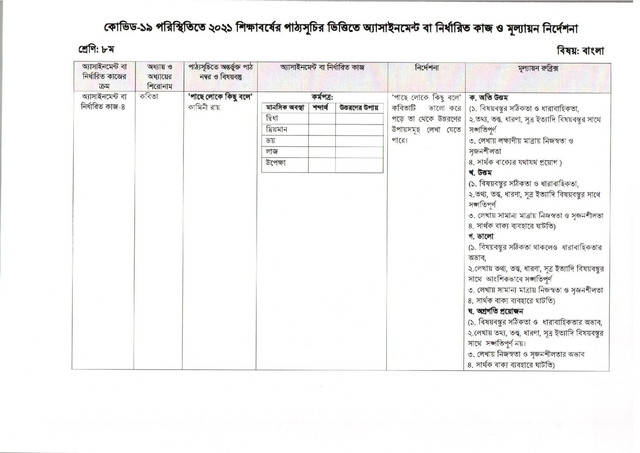Assignment

# Class 8 Assignment 13th week 2023 (Bangla and Math)

Assignments of students will start from 26th August 2023 and in the light of the short syllabus prepared for 8th class students in 2023, the instructions and assignments mentioned in the 13th week assignment question paper will be completed and the subject of the studying institution will be submitted to the teacher. [ বাংলায় দেখুন ]

## Class 8 13th Week Bangla AssignmentSubject: Bengali, Assignment Number: 04

The title of the assignment and the chapter: Poetry

Learning Outcomes / Content:

Kamini Roy;

Assignment:

Worksheet:

Mental state is the way to pass the semantics
Hesitation
Dreamy
Fear
Shame
Ignore
Instructions (signal / step / circumference):

Ways of overcoming it can be written by reading the poem ‘leche lake kishi baye’ well;

## Class 8 13th Week Math AssignmentSubject: Mathematics, Assignment Number: 03

Assignment and Chapter Title: Chapter-IV; Algebraic formulas and applications;

Learning Outcomes / Content:

4.1 Algebraic formulas 4.2 Cube formulas and conclusions 4.3 Two more formulas associated with cubes 4.4 Analysis of product 4.5 x ^ 2 + px + q is the product of the size;

4.6 ax ^ 2 + px + c is the product of size 4.6 is the algebraic sum c. Sa, Gu and L. Sa. Gu 4.6.1 The highest common factor (GCG) 4.6.2 The lowest common factor.

Assignment:

A = x ^ 2 – 2x + 1,

B = 2x ^ 2 – 6x + 4;

C = x ^ 2 + 4x – 5

A. If A = 0, x ^ 3 + 1 / x ^ 3 = how much?

B. A, B and C are divisible by which largest number?

C. Which smallest sum can be divided by A, B and C?

Instructions (signal / step / circumference):

The algebraic cumulative formulas discussed in the 4th chapter of the Mathematics textbook will solve the problems with the concept of determining the intermediate product, L.S.G. and G.S.G.

For the convenience of the eighth student of 2023, the assignment was given in the form of a PDF in one page of the 13th week published for the students;

Download the 13th week assignment PDF on one page by clicking on the desired button below;